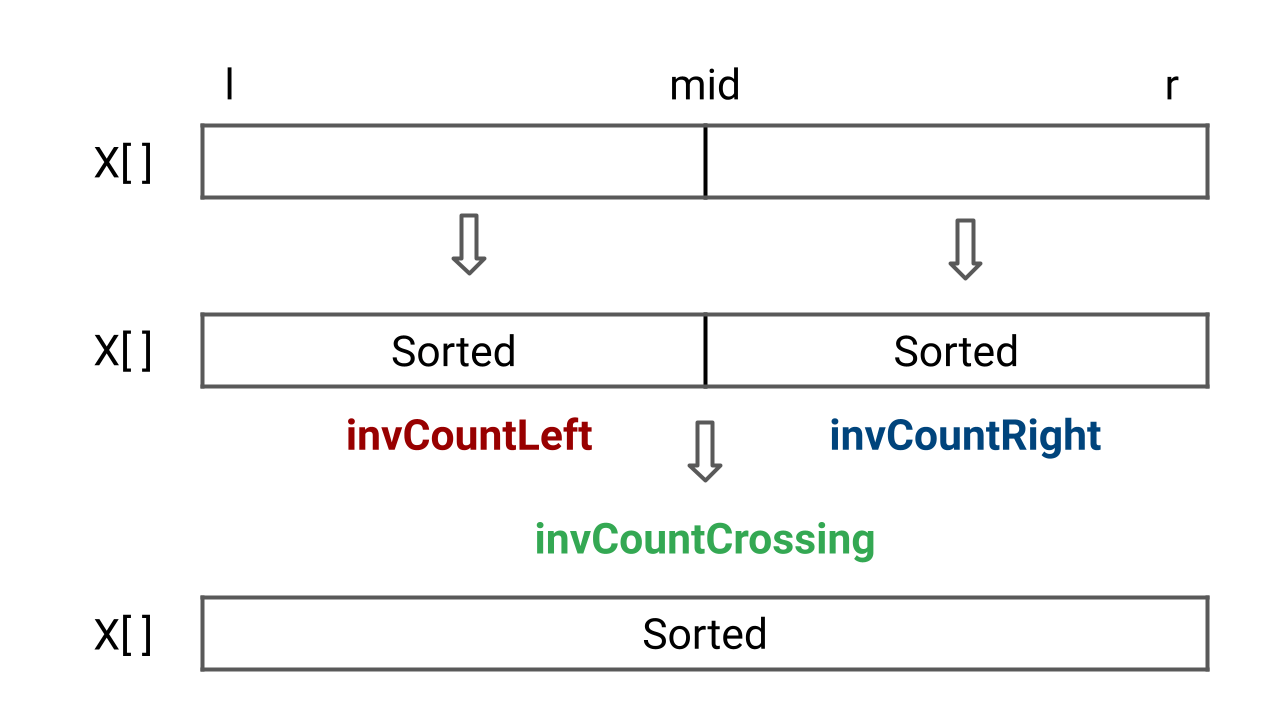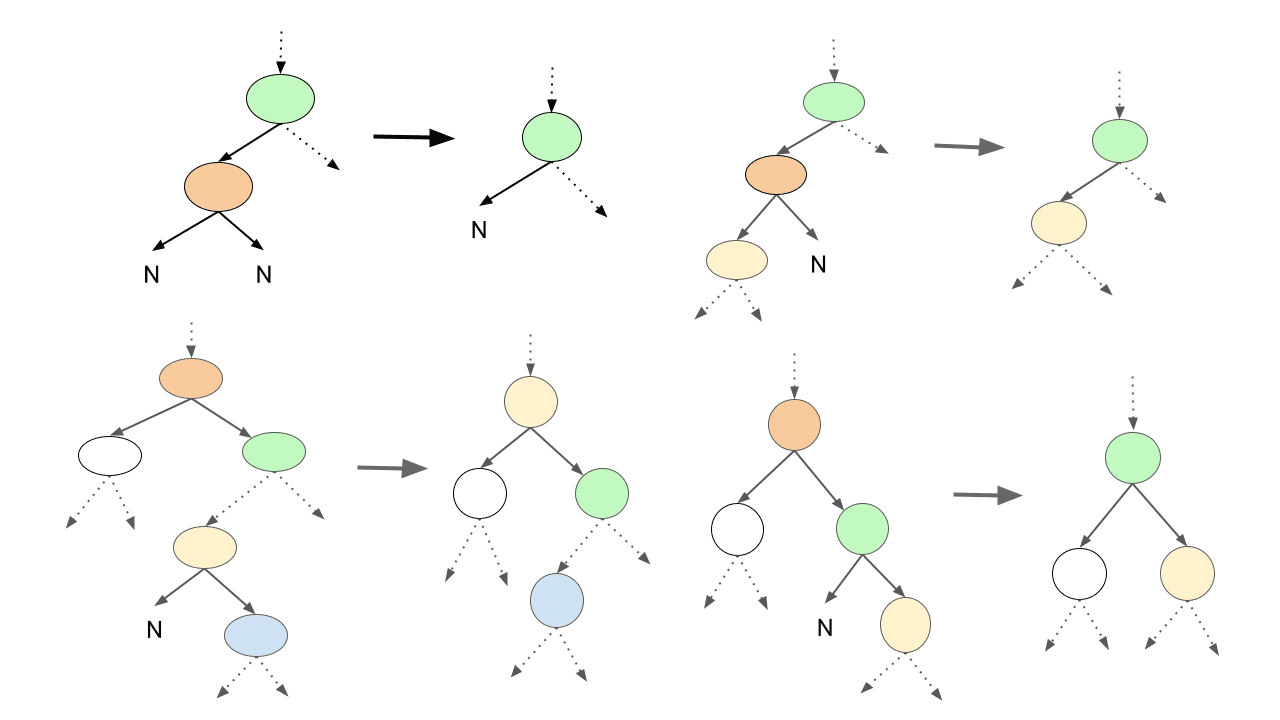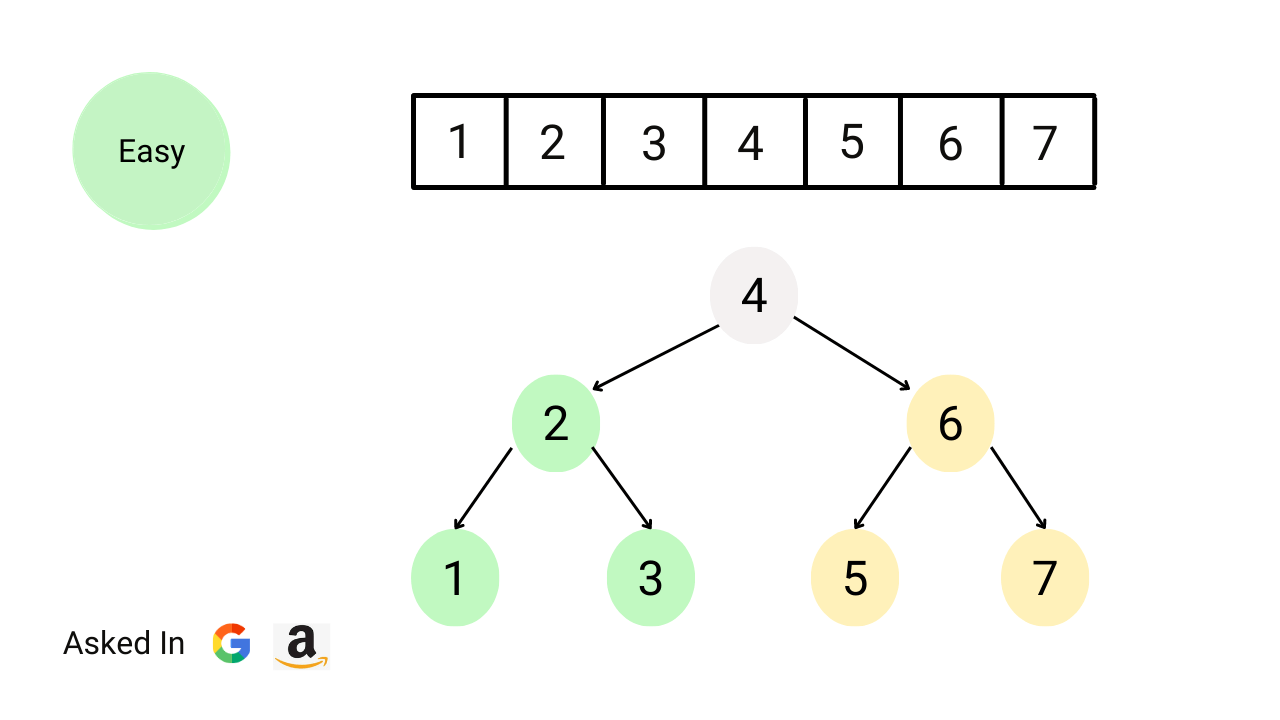# recursionDivide and Conquer Algorithm

In data structures and algorithms, Divide and Conquer is a recursive problem-solving approach that divides the problem into smaller subproblems, recursively solves each subproblem, and combines the subproblem's solutions to get the solution of the original problem. So, there are four steps of the divide and conquer method: divide, conquer, combine and base case.Median of Two Sorted Arrays

There are two sorted arrays A and B of size n each, write a program to find the median of these two sorted arrays obtained after merging (the new merged array will be an array of length 2n). The median of a sorted array of size n is defined as the middle element when n is odd and the average of the middle two elements when n is even.Flood Fill Algorithm

The flood fill problem is a way to fill a region of connected pixels with a new colour, starting from a given pixel in an image. You are given an image represented by an m x n grid of integers, where the image[i] represents the pixel value at position (i, j). You are also given the coordinates of a starting pixel (x, y) and a new colour to use for the flood fill operation.Generating all K-combinations

Given two numbers, n and K, write a program to find all possible combinations of K numbers from 1 to n. You may return the answer in any order. Note: This is an excellent problem for learning problem-solving using the inclusion and exclusion principle of combinatorics and backtracking. We can apply similar ideas to solve other problems.Divide and Conquer vs Dynamic Programming

Divide and conquer and dynamic programming differ in terms of implementation, analysis, and the nature of the subproblems. Divide and conquer divides the problem into independent subproblems, solves them recursively, and combines them to get the solution. In dynamic programming, problems are divided into dependent subproblems and solved in an order to get the solution.Count Inversions of an Array

Given an array of n integers, write a program to find the total number of inversion counts. An inversion occurs when there are two elements in the array such that i < j and X[i] > X[j]. Here, the pair (i, j) is called an inversion of X[], and the inversion count represents the count of such inversions present in the array.Quicksort Algorithm

Quicksort algorithm is often the best choice for sorting because it works efficiently on average O(nlogn) time complexity. It is also one of the best algorithms to learn divide and conquer approach. In this blog, you will learn: 1) How quick sort works? 2) How to choose a good pivot? 3) Best, worst, and average-case analysis 4) Space complexity and properties of quicksort.Merge Sort Algorithm

Merge sort is one of the fastest comparison-based sorting algorithms, which works on the idea of a divide and conquer approach. The worst and best-case time complexity of the merge sort is O(nlogn), and the space complexity is O(n). It is also one of the best algorithms for sorting linked lists and learning the design and analysis of recursive algorithms.Binary Search Algorithm

Binary search is an efficient algorithm for searching a value in a sorted array using the divide and conquer idea. It compares the target value with the value at the mid-index and repeatedly reduces the search interval by half. The search continues until the value is found or the subarray size gets reduced to 0. The time complexity of the binary search is O(log n).Delete Node in a Binary Search Tree

Given a binary search tree and a key value. Write a program to delete the given key from the binary search tree and return the updated root node. This is an excellent problem to learn pointer manipulation in binary trees and problem-solving using both iterative and recursive approaches.Recursion vs Iteration Comparison

Understanding the difference between iteration and recursion is important for learning various solving techniques in programming. If we compare iterative and recursive approaches, one thing is common: Repeated execution of instructions until the task is done. But there are differences in terms of implementation, execution, analysis, thought process, etc.Convert sorted array to balanced BST

Write a program to convert a sorted array of integers into a balanced binary search tree. Each node in the tree must follow the BST property, and the height of the left and right subtrees should be as close to equal as possible. Note: This is an excellent problem to learn problem-solving using the divide and conquer approach.Sort a Stack

Given a stack, write a program to sort a stack in ascending order. We are not allowed to make assumptions about how the stack is implemented. The only functions to be used are push(s, x), pop(s), top(s), isEmpty(s). In this blog, we have discussed two approaches: 1) Sorting stack using temporary stack 2) Sorting stack using recursion.Write a program to find intersection of two sorted linked lists and return the head pointer of the new linked list. Here head pointers of both sorted linked lists are given as input and we shouldn’t do any changes in the structure to generate the output. Note: This is an excellent problem to learn problem-solving using recursion and two pointers in a linked list.Spiral Traversal of Matrix

Given a 2-dimensional matrix, write a program to print matrix elements in spiral order. We can imagine spiral traversal as an ordered set of matrix segments with horizontal and vertical boundaries, where both boundaries are reduced by one at each step. Note: This is an excellent problem to learn problem-solving using iteration and recursion.A head pointer of a singly linked list is given, write a program to reverse linked list and return the head pointer of the reversed list. We need to reverse the list by changing the links between nodes. Note: This is an excellent question to learn problem-solving using both iteration (Three-pointers) and recursion (Decrease and conquer approach).Preorder, Inorder and Postorder Traversal using Recursion

There are three types of recursive tree traversals: preorder, inorder and postorder. This classification is based on the visit sequence of root node 1) Preorder traversal: root is visited first 2) Inorder traversal: root is visited after left subtree 3) Postorder traversal: root is visited last. These are also called depth first search or DFS traversal.Recursion Explained: What is Recursion in Programming?

Recursion means solving the problem using the solution of smaller sub-problems. This blog will explain these critical concepts: 1) What is recursion? 1) How recursion works in programming? 2) Advantages and disadvantages of recursion 3) Steps to solve problems using recursion? 4) Difference between recursion and iteration? Etc.Analysis of Recursion in Data Structures and Algorithms

In DSA, learning the time complexity analysis of recursion is one of the critical steps in mastering problem-solving using recursion. In this blog, we will discuss: 1) How to write recurrence relations of recursive algorithms with various examples 2) Steps to analyze the time complexity of recursion 3) Popular methods of analysis like the recursion tree method and the master theorem.Segment Trees (Point and Range Update, Lazy Propagation)

In this blog, we will learn how to use segment trees for efficient point and range updates. For point update query, update(idx, val), we need to increment element at index idx from the original array by val, i.e. arr[idx] = arr[idx] + val. For range update query, update(l, r, val), we increment all elements from index l to r from the original array by val.Segment Tree (Build and Range Query Operations)

A segment tree is a binary tree data structure such that each node stores information about a range. We use segment trees to efficiently answer multiple range queries on an array like range minimum, range maximum, range sum, etc. Also, it allows us to modify the array by replacing an element or an entire range of elements in logarithmic time.Swap List Nodes in Pairs

Given the head pointer of a singly linked list, write a program to swap nodes in pairs and return the head of the modified linked list. If the number of nodes is odd, then we need to pairwise swap all nodes except the last node. Note: This is an excellent problem to learn problem solving using both iteration and recursion in a linked list.